Electric potential energy

1. Introduction

• We already have an introduction of work and energy while studying mechanics.
• We know that central forces are conservative in nature i.e., work done on any particle moving under the influence of consrevative forces does not depend on path taken by the particle but depends on initial and final positions of the particle.
• Electrostatic force given by Coulumb's law is also a central force like gravitational force and is conservative in nature.
• For conservative forces, work done on particle undergoing displacement can be expressed in terms of potential energy function.
• In this chapter we will apply work and energy considerations to the electric field and would develop the concept of electric potential energy and electric potential.

2. Electric potential energy

• Conside a system of two point charges in which positive test charge q' moves in the field produced by stationary point charge q shown below in the figure.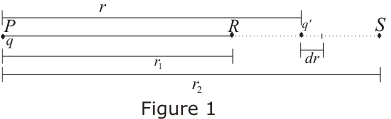• Charge q is fixed at point P and is displaced from point R to S along a radial line PRS shown in the figure.
• Let r1 be the distance between points P and R and r2 be the distance between P and S.
• Magnitude of force on positive test charge as given by Coulumb's law is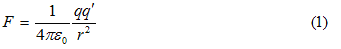• If q' moves towards S through a small displacement dr then work done by this force in making the small displacement dr is
dW=F·dr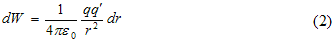• Total work done by this force as test charge moves from point R to S i.e., from r1 to r2 is,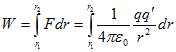or• Thus for this particular path work done on test charge q' depends on end points not on the path taken.
• Work done W in moving the test charge q' from point R to S is equal to the change in potential energy in moving the test charge q' from point R to S. Thus,
W=U(r1)-U(r2)                                          (4)
where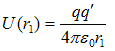is the potential energy of test charge q' when it is at point R and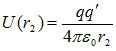is the potential energy of test charge q' when it is at point S.
• Thus potential energy of test charge q' at any distance r from charge q is given by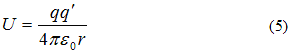Equation 5 gives the electric potential energy of a pair of charges which depends on the separation between the charges not on the location of charged particles.
• If we bring the test charge q' from a very large distance such that r2=∞ to some distance r1=r then we must do work against electric forces which is equal to increase in potential energy as given by equation 5.
• Thus potential energy of a test charge at any point in the electric field is the work done against the electric forces to bring the charge from infinity to point under consideration.